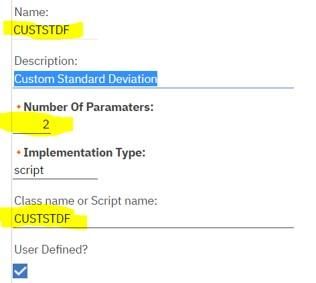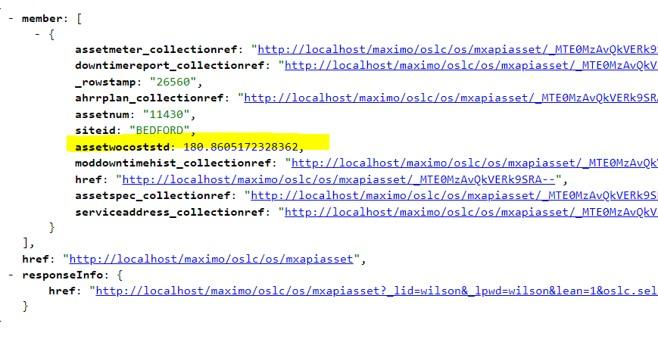# Formula functions

Maximo Formulas provide an Excel-like expression library to calculate numeric values from MBOs. The framework provides many standard functions in the default version. Scripting framework provides customers with a way to create custom functions to extend the library of built-in functions. The following example shows the setup steps to add the standard deviation function using scripting:

1. Write the formula script code (python sample). This leverages the apache math library to calculate the standard deviation.
```from org.apache.commons.math3.stat.descriptive import DescriptiveStatisticsfrom java.lang import Math
relation = ctx.getExpression().getCalculatedValue(params)attr = ctx.getExpression().getCalculatedValue(params)dstats = DescriptiveStatistics()workOrders = mbo.getMboSet(relation)wo = workOrders.moveFirst()while wo is not None:```

The following list of implicit variables is supported by this script point:

VariablePurpose
expAn instance of com.ibm.tivoli.maximo.expression.Expression which is a handle to the parsed formula expression instance.
ctxAn instance of the com.ibm.tivoli.maximo.expression.ScriptFunction function.
mboThe MBO in context for the Formula.
paramsThe input parameters.
1. Add a new Formula function with the implementation type as “script” and the Class name or script name as the name of the script (in uppercase).1. Add or modify Object or Attribute Formula. The formula for this sample is on Asset object.A way to test this would be to make the following REST API call:

`GET /oslc/os/mxapiasset?lean=1&oslc.select=assetnum,siteid,exp.assetwocoststd&oslc.where=assetnum="11430"`

The following graphic shows a sample result: